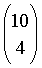SEARCH HOMEMath Central Quandaries & QueriesQuestion from jeremy: Here's my question... I've been playing the lottery, I know what a waste of time. I would like to know how many 4 number combinations can be derived from a set of 10 numbers, when no number can be repeated in the present string of 4 numbers being selected. e.g. Lets say my numbers are: 1 - 10 I could select: 1, 2, 3, 4 (as one of my sets) and 1, 2, 3, 5, (as another) I couldn't select: 1, 1, 2, 3 (I can't repeat any numbers in a group of four)Jeremy,

Well, Jeremy, since you have so much spare time <grin> you can work it out.

1. How many choices do you have for your _first_ number? (this one is easy!)

2. Once the first number is picked, how many choices do you have for the second number? So how many ordered pairs (without repetition) are there from 10 choices?

3. Once the first two numbers are picked, how many choices do you have for the third number? How many ordered triples are there?

4. Once the first three numbers are picked, how many choices do you have for the last number? How many ordered quadruples are there?

5. Now this last number is too big to be the answer because you will have counted 1234, 2134, ... separately, and all of these represent the unordered quadruple {1,2,3,4}. How many times has each quadruple been counted? Divide to get the answer.

Good Hunting!
RD

Jeremy,

Mathematicians call these combinations. In mathematical language you are looking for the number of combinations of ten things taken four at a time and it's writtenor 10C4. A way to calculate this number can be found in Penny's response to an earlier question. If you simply want the numerical answer you can type 10 choose 4 into the Google search engine and Google will respond with 10 choose 4 = 210.

HarleyMath Central is supported by the University of Regina and The Pacific Institute for the Mathematical Sciences.